# 24. Appendix: Natural Deduction Rules¶

Implication: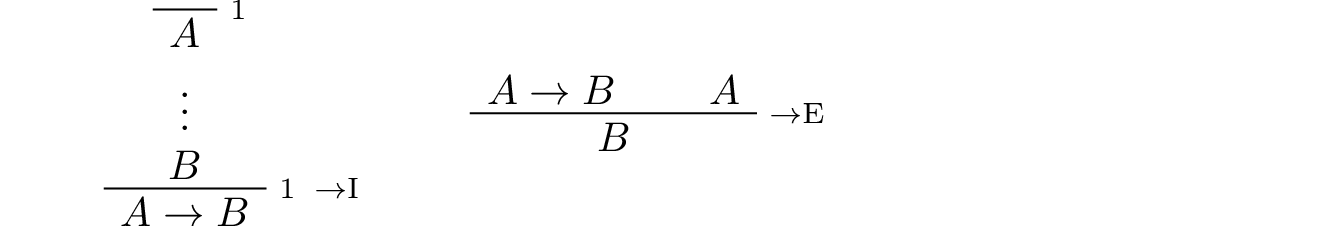Conjunction:Negation: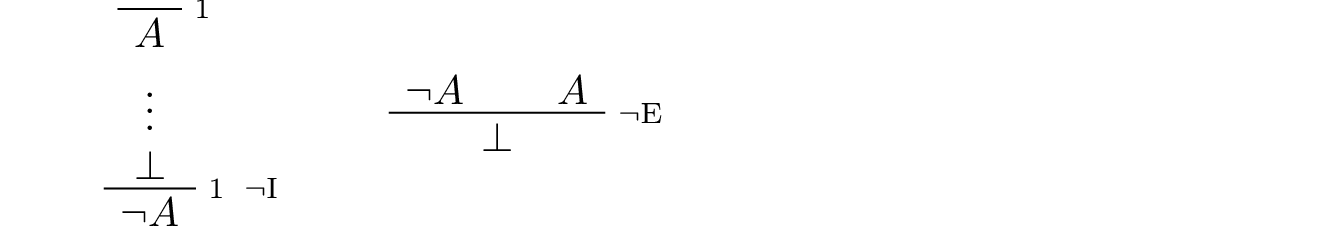Disjunction: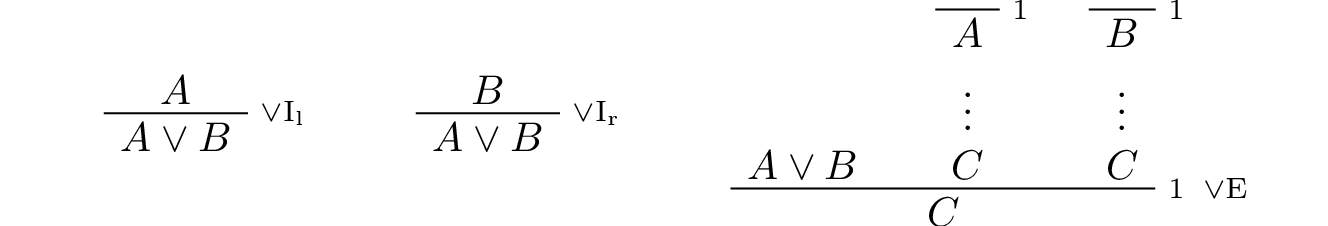Truth and falsity:Bi-implication: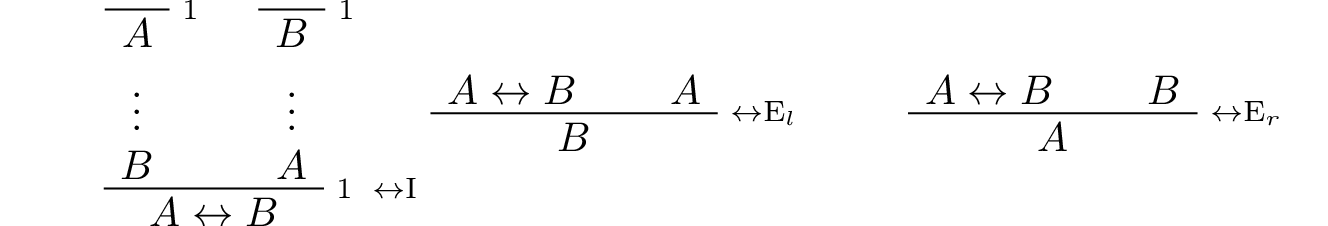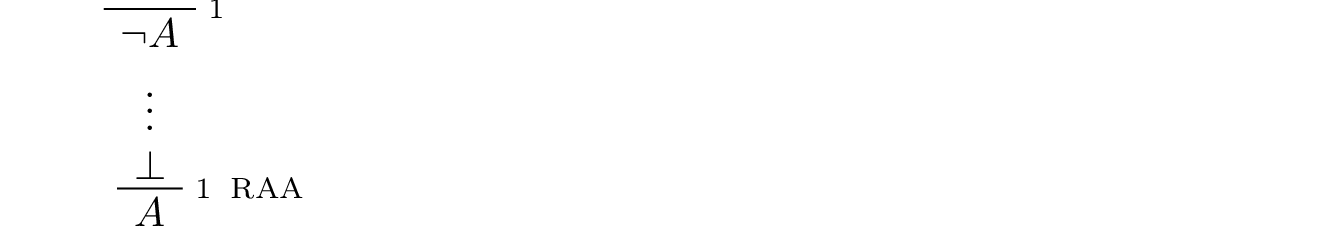The universal quantifier:In the introduction rule, $$x$$ should not be free in any uncanceled hypothesis. In the elimination rule, $$t$$ can be any term that does not clash with any of the bound variables in $$A$$.

The existential quantifier: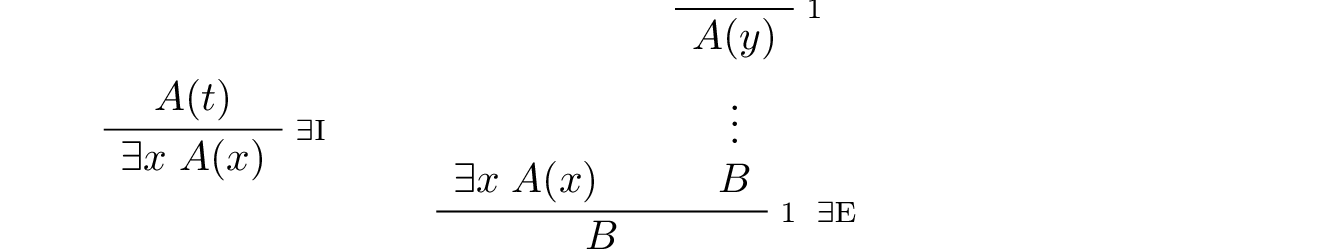In the introduction rule, $$t$$ can be any term that does not clash with any of the bound variables in $$A$$. In the elimination rule, $$y$$ should not be free in $$B$$ or any uncanceled hypothesis.

Equality:Strictly speaking, only $$\mathrm{refl}$$ and the second substitution rule are necessary. The others can be derived from them.